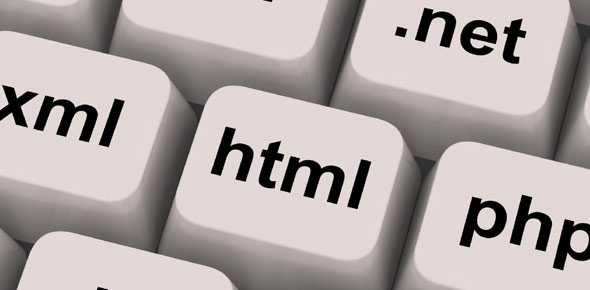# Data Types Trivia Quiz

9 Questions | Attempts: 129Settings• 1.
Predict the output of following program. Assume that the numbers are stored in 2's complement form.#include<stdio.h>  int  main()  {     unsigned int x = -1;     int y = ~0;     if(x == y)        printf("same");     else       printf("not same");     return 0;  }
• A.

Same

• B.

Not Same

• 2.
Which of the following is not a valid declaration in C?1. short int x; 2. signed short x; 3. short x; 4. unsigned short x;
• A.

3 and 4

• B.

2

• C.

1

• D.

All are valid

• 3.
Predict the output#include <stdio.h> int main() {    float c = 5.0;    printf ("Temperature in Fahrenheit is %.2f", (9/5)*c + 32);    return 0; }
• A.

Temperature in Fahrenheit is 41.00

• B.

Temperature in Fahrenheit is 37.00

• C.

Temperature in Fahrenheit is 0.00

• D.

Compiler Error

• 4.
Predict the output of following C program#include <stdio.h> int main() {     char a = '\012';      printf("%d", a);      return 0; }
• A.

Compiler error

• B.

12

• C.

10

• D.

Empty

• 5.
In C, sizes of an integer and a pointer must be same.
• A.

True

• B.

False

• 6.
Output?int main() {     void *vptr, v;     v = 0;     vptr = &v;     printf("%v", *vptr);     getchar();     return 0; }
• A.

0

• B.

Compiler error

• C.

Garbage value

• 7.
Assume that the size of char is 1 byte and negatives are stored in 2's complement form#include<stdio.h> int main() {     char c = 125;     c = c+10;     printf("%d", c);     return 0; }
• A.

135

• B.

+INF

• C.

-121

• D.

-8

• 8.
#include <stdio.h> int main() {     if (sizeof(int) > -1)         printf("Yes");     else         printf("No");     return 0; }
• A.

Yes

• B.

No

• C.

Compiler error

• D.

Run time error

• 9.
Output of following program?#include<stdio.h> int main() {     float x = 0.1;     if ( x == 0.1 )         printf("IF");     else if (x == 0.1f)         printf("ELSE IF");     else         printf("ELSE"); }
• A.

ELSE IF

• B.

IF

• C.

ELSE

## Related TopicsBack to top
×

Wait!
Here's an interesting quiz for you.